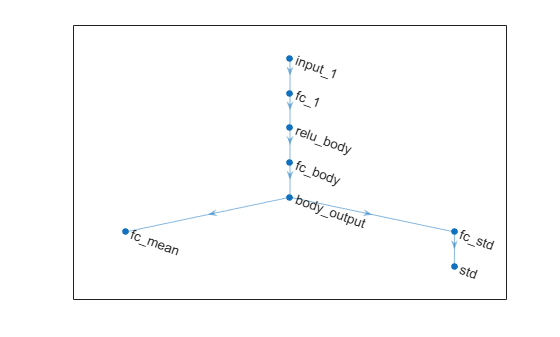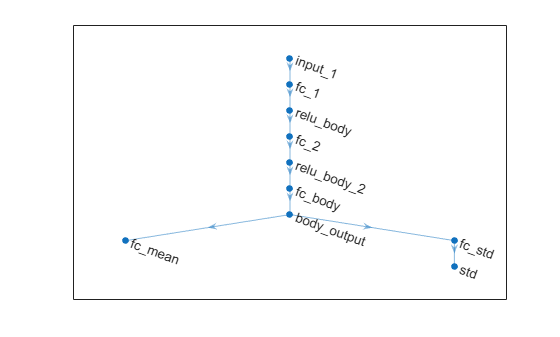# getCritic

Get critic from reinforcement learning agent

## Syntax

``critic = getCritic(agent)``

## Description

example

````critic = getCritic(agent)` returns the critic object from the specified reinforcement learning agent.```

## Examples

collapse all

Assume that you have an existing trained reinforcement learning agent. For this example, load the trained agent from Train DDPG Agent to Control Double Integrator System.

`load('DoubleIntegDDPG.mat','agent') `

Obtain the critic function approximator from the agent.

`critic = getCritic(agent);`

Obtain the learnable parameters from the critic.

`params = getLearnableParameters(critic)`
```params=2×1 cell array {[-5.0077 -1.5619 -0.3475 -0.0961 -0.0455 -0.0026]} {[ 0]} ```

Modify the parameter values. For this example, simply multiply all of the parameters by `2`.

`modifiedParams = cellfun(@(x) x*2,params,'UniformOutput',false);`

Set the parameter values of the critic to the new modified values.

`critic = setLearnableParameters(critic,modifiedParams);`

Set the critic in the agent to the new modified critic.

`setCritic(agent,critic);`

Display the new parameter values.

`getLearnableParameters(getCritic(agent))`
```ans=2×1 cell array {[-10.0154 -3.1238 -0.6950 -0.1922 -0.0911 -0.0052]} {[ 0]} ```

Create an environment with a continuous action space and obtain its observation and action specifications. For this example, load the environment used in the example Train DDPG Agent to Control Double Integrator System.

`env = rlPredefinedEnv("DoubleIntegrator-Continuous");`

Obtain observation and action specifications.

```obsInfo = getObservationInfo(env); actInfo = getActionInfo(env);```

Create a PPO agent from the environment observation and action specifications. This agent uses default deep neural networks for its actor and critic.

`agent = rlPPOAgent(obsInfo,actInfo);`

To modify the deep neural networks within a reinforcement learning agent, you must first extract the actor and critic function approximators.

```actor = getActor(agent); critic = getCritic(agent);```

Extract the deep neural networks from both the actor and critic function approximators.

```actorNet = getModel(actor); criticNet = getModel(critic);```

The networks are `dlnetwork` objects. To view them using the `plot` function, you must convert them to `layerGraph` objects.

For example, view the actor network.

`plot(layerGraph(actorNet))`To validate a network, use `analyzeNetwork`. For example, validate the critic network.

```analyzeNetwork(criticNet) ```

You can modify the actor and critic networks and save them back to the agent. To modify the networks, you can use the Deep Network Designer app. To open the app for each network, use the following commands.

```deepNetworkDesigner(layerGraph(criticNet)) deepNetworkDesigner(layerGraph(actorNet)) ```

In Deep Network Designer, modify the networks. For example, you can add additional layers to your network. When you modify the networks, do not change the input and output layers of the networks returned by `getModel`. For more information on building networks, see Build Networks with Deep Network Designer.

To validate the modified network in Deep Network Designer, you must click on Analyze for dlnetwork, under the Analysis section. To export the modified network structures to the MATLAB® workspace, generate code for creating the new networks and run this code from the command line. Do not use the exporting option in Deep Network Designer. For an example that shows how to generate and run code, see Create Agent Using Deep Network Designer and Train Using Image Observations.

For this example, the code for creating the modified actor and critic networks is in the `createModifiedNetworks` helper script.

`createModifiedNetworks`

Each of the modified networks includes an additional `fullyConnectedLayer` and `reluLayer` in their main common path. View the modified actor network.

`plot(layerGraph(modifiedActorNet))`After exporting the networks, insert the networks into the actor and critic function approximators.

```actor = setModel(actor,modifiedActorNet); critic = setModel(critic,modifiedCriticNet);```

Finally, insert the modified actor and critic function approximators into the actor and critic objects.

```agent = setActor(agent,actor); agent = setCritic(agent,critic);```

collapse all

## Output Arguments

collapse all

Critic object, returned as one of the following:

• `rlValueFunction` object — Returned when `agent` is an `rlACAgent`, `rlPGAgent`, or `rlPPOAgent` object.

• `rlQValueFunction` object — Returned when `agent` is an `rlQAgent`, `rlSARSAAgent`, `rlDQNAgent`, `rlDDPGAgent`, or `rlTD3Agent` object with a single critic.

• `rlVectorQValueFunction` object — Returned when `agent` is an `rlQAgent`, `rlSARSAAgent`, `rlDQNAgent`, object with a discrete action space, vector Q-value function critic.

• Two-element row vector of `rlQValueFunction` objects — Returned when `agent` is an `rlTD3Agent` or `rlSACAgent` object with two critics.

## Version History

Introduced in R2019a